# Markov Chain Monte Carol (MCMC)

Posted by Gwan Siu on April 5, 2019

## 1. Monte Carlo methods

### 1.1 Monte Carlo methods are algorithm that:

• Generate samples from a given probability distribution $p(x)$.
• Estimate expectations of functions $\mathbb{E}[f(x)]$ under a distribution $p(x)$

### 1.2 Why Monte Carlo useful?

• Can use samples of $p(x)$ to approximate $p(x)$ itself, allow us to do graphical model inference when we can’t compute $p(x)$.

• Expectation $\mathbb{E}[f(x)]$ reveal interesting properties about $p(x)$, e.g. means and variances.

### 1.3 Limitation of Monte Carlo

• Direct sampling
• Hard to get rare events in high-dimensional spaces.
• Infesible for MRFs, unless we know the normalizer $Z$.
• Rejection sampling, Important sampling
• Do not work well if the proposal $Q(x)$ is very different from $P(x)$.
• Construct a $Q(x)$ similar to $P(x)$ can be diffuclt
• Requires knowledge of analytical form of $P(x)$ - but if we had that, we wouldn’t even need to sample
• Intuition:Instead of a fixed proposal $Q(x)$, use a adaptive proposal.

## 2. Markov Chain Monte Carol(MCMC)

MCMC algorithms provides adaptive proposals probability.

• Instead of $Q(x^{\prime})$, use $Q(x^{\prime}\vert x)$ where $x^{\prime}$ is the new state being sampled, and $x$ is the previous sample.

• As $x$ changes, $Q(x^{\prime}\vert x) can also change (as a function of$x^{\prime}$).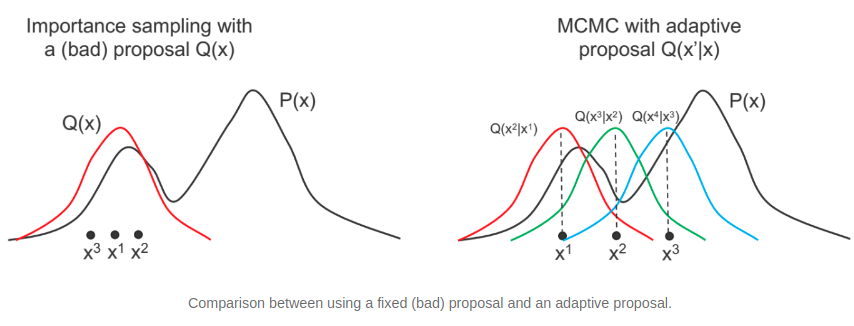### 2.1 What’s the Markov Chain (Definition:) A Markov Chain is a stochastic process that a sequence of random variable$x_{1},x_{2},…,x_{n}$with markov property $\begin{equation} T(x_{n}=x\vert x_{1},x_{2},...,x_{n-1})=T(x_{n}=x\vert x_{n-1}) \end{equation}$ the probability of the next step only depends on the current state. • Random variable$x_{i}$can be vectors. We define$x_{i}$to be the$t-$th sample of all variables in a graphical model. •$x_{i}$represents the entire state of the graphical model at time$t$. We study homogenous Markov Chains, in which the transition kernel$P(x_{n}=x\vert x_{n-1})$is fixed with time. To emphasize this, we will call the kernel$T(x^{\prime}\vert x)$, where$x$is the previous state and$x^{\prime}$is the next state. ### 2.2 Markov Chains Concepts • Probability distribution over states:$\pi^{(t)}$is a distribution over the state of system$x$, at time$t$. • When dealing with$MCs$, the system can’t be considered as being in one state, but having a distribution over states. • For graphical models,$x$represent all variabls. • Transition: recall that states transition from$x^{(t)}$to$x^{(t+1)}$according to the transition kernel$T(x^{\prime}\vert x)$. • We can also define the entire distribution:$\pi^{(t+1)}(x^{\prime})=\sum_{x}\pi^{(t)}(x)T(x^{\prime}\vert x)$. • At time$t$, state$x$has probability mass$x^{(t)}(x)$. The transition probability redistributes this mass to other state$x^{\prime}$• Stationary distributions:$\pi^{(t)}(x)$is stationary if it does not change under the transition kernel:$\pi(x^{\prime})=\sum_{x}\pi(x)T(x^{\prime}\vert x)$, for all$x^{\prime}$. • Irreducible: an MC is irreducible if you can get from any state$x$to any other state$x^{\prime}$with probability$> 0$in a finite number of steps, i.e. there are no unreachable parts of the state space. • Aperiodic: an MC is aperiodic if you can return to any state$x$at any time. (There are no deterministic loops). Periodic MCs have states that need$\geq 2$time steps to return to (cycles). • Reversible (detailed balance): an MC is reversible if there exists a distribution$\pi(x)$such that the detailed balance condition is satisfied:$\pi(x^{\prime})T(x\vert x^{\prime})=\pi(x)T(x^{\prime}\vert x)$with probability of$x^{\prime}\rightarrow x$is the same as$x\rightarrow x^{\prime}$. • Reversible MCs always have a stationary distribution. Proof: $\begin{equation} \begin{split} \pi(x^{\prime})T(x\vert x^{\prime}) &= \pi(x)T(x^{\prime}\vert x) \\ \sum_{x}\pi(x^{\prime})T(x\vert x^{\prime}) &= \sum_{x}\pi(x)T(x^{\prime}\vert x) \\ \pi(x^{\prime})\sum_{x}T(x\vert x^{\prime}) &= \sum_{x}\pi(x)T(x^{\prime}\vert x) \\ \pi(x^{\prime}) &= \sum_{x} \pi(x)T(x^{\prime}\vert x) \end{split} \end{equation}$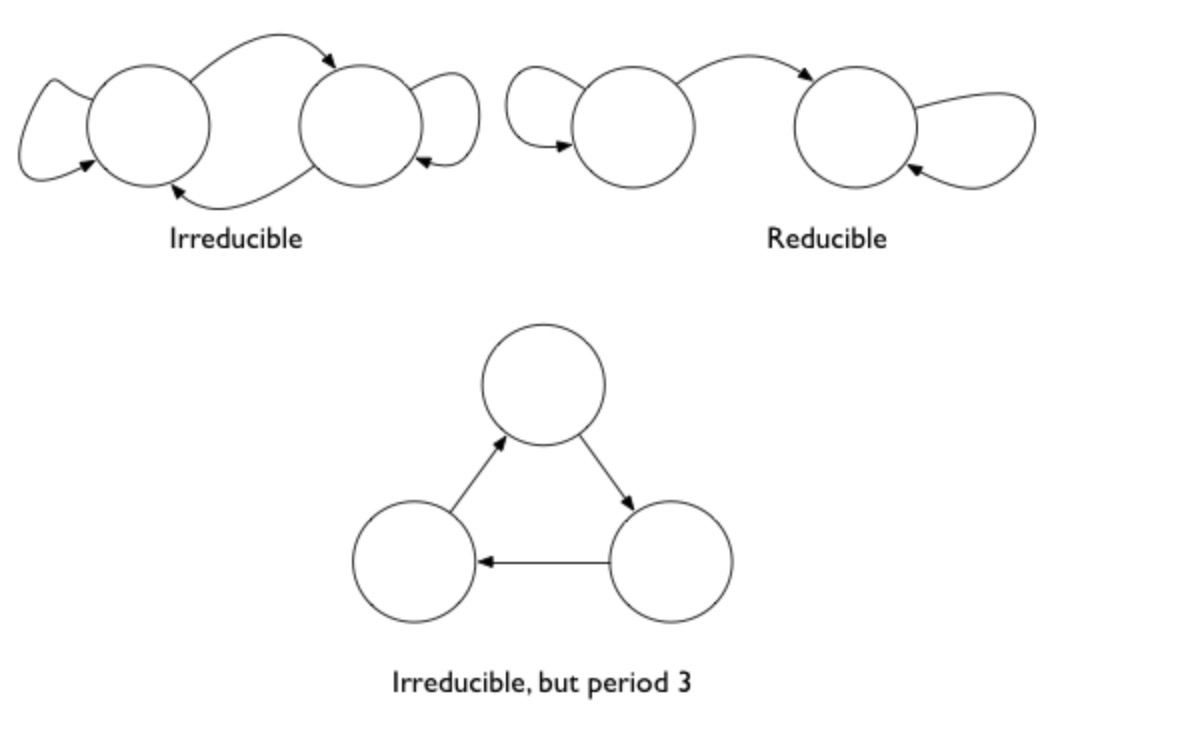(摘抄慕课网笔记)在满足一定条件的情况下，马尔可夫过程将收敛至一个均衡。这是一个统计均衡，在每种状态下的概率是固定不变的，但事物将依旧在各个状态间转移。 马尔可夫过程收敛到均衡的四个条件： 一、可能的状态数量是有限的。 二、转移概率固定不变。 三、从任意一个状态能够变到任意其他一个状态。有可能不是从状态A直接变到状态C，而是先变到状态B再变到C，但只要有路径从状态A变成状态C就行。 四、过程不是简单循环。比如不能是从全A变到全B，然后又自动从全B变到全A。 马尔可夫收敛定理（Markov Convergence Theorem）：如果满足上述四个条件，一个马尔科夫过程将收敛到一个均衡状态，且此均衡唯一。 只要转移概率不变，那么初始状态、历史过程、中途干预都不重要，最后必将达到那个唯一的均衡。换句话说，马尔科夫链最后达到的均衡与初始状态，转移过程以及中途干预无关。  import scipy.stats as st def target(lik, prior, n, h, theta): if theta < 0 or theta > 1: return 0 else: return lik(n, theta).pmf(h)*prior.pdf(theta) def mh_coin(niters, n, h, theta, lik, prior, sigma): samples = [theta] while len(samples) < niters: theta_p = theta + st.norm(0, sigma).rvs() rho = min(1, target(lik, prior, n, h, theta_p)/target(lik, prior, n, h, theta )) u = np.random.uniform() if u < rho: theta = theta_p samples.append(theta) return samples n = 100 h = 61 lik = st.binom prior = st.beta(10, 10) sigma = 0.05 niters = 100 sampless = [mh_coin(niters, n, h, theta, lik, prior, sigma) for theta in np.arange(0.1, 1, 0.2)] # Convergence of multiple chains for samples in sampless: plt.plot(samples, '-o') plt.xlim([0, niters]) plt.ylim([0, 1]);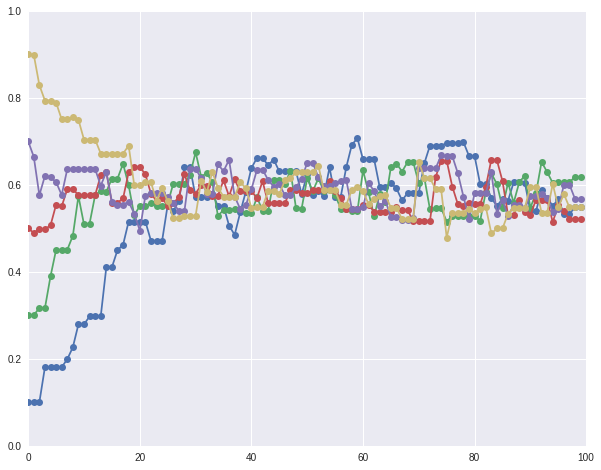### 2.3 Metropolis Hasting Algorithm(MH Algorithm) the idea of MH algorithm 1. Draws a sample$x^{\prime}$from$Q(x^{\prime}\vert x)$, where$x$is the previous sample. 2. The new sample$x^{\prime}$is accepted with the probability$A(x^{\prime}\vert x)=\min (1, \frac{P(x^{\prime})Q(x\vert x^{\prime})}{P(x)Q(x^{\prime}\vert x)})$•$A(x^{\prime}\vert x)$is like a ratio of importance sampling weights •$\frac{P(x^{\prime})}{Q(x^{\prime}\vert x)}$is the important weight for$x^{\prime}$,$\frac{P(x)}{Q(x\vert x^{\prime})}$is the important weight for$x$. • We divide the important weight for$x^{\prime}$by that of$x$. • Notice that we only need to compute$\frac{P(x^{\prime})}{P(x)}$rather than$P(x^{\prime})$or$P(x)$separately, so we don’t need to know the normalizer. •$A(x^{\prime}\vert x)$ensures that, after sufficiently many draws, our samples will come from the true distribution$P(x).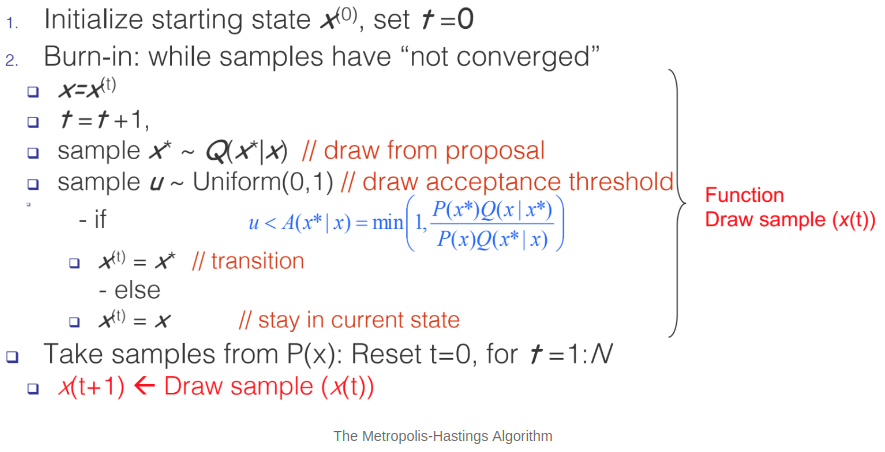To verify the detail balance condition: \begin{aligned} s(x^{*})q(x^{*}\vert x)\alpha(x^{*},x) &= s(x^{*})q(x^{*}\vert x)\min(1,\frac{s(x^{*})q(x\vert x^{*})}{s(x)q(x^{*}\vert x)}) \\ &= \min(s(x)q(x^{*}\vert x), s(x^{*})q(x\vert x^{*})) \\ &= s(x)q(x\vert x^{*})\min(1,\frac{s(x)q(x^{*}\vert x)}{s(x^{*})q(x\vert x^{*})}) \\ &= s(x)q(x\vert x^{*})\alpha(x,x^{*}) \end{aligned} (why do we need Metropolis Hasting Algorithm?) We’ve learnt how to do the inverse transform and how to use rejection sampling with a majority function. So why do we use these methods to sample a ditribution? ** inefficient as dimensions increased.** In other words, dimension curse. How do we understand this point? In generally, we want to calculate the expectation of distribution as sample average, however, as dimension of space increased, majorizing in multiple dimensions can have us spending a lot of time in tail dimension because you leave more and more space out. If inverse tranform and reject sampling methods are adopted, then it will boost inefficient. In multiple dimensions, volumns get smaller and smaller, that’s the curse of dimension. This concept can be shown as: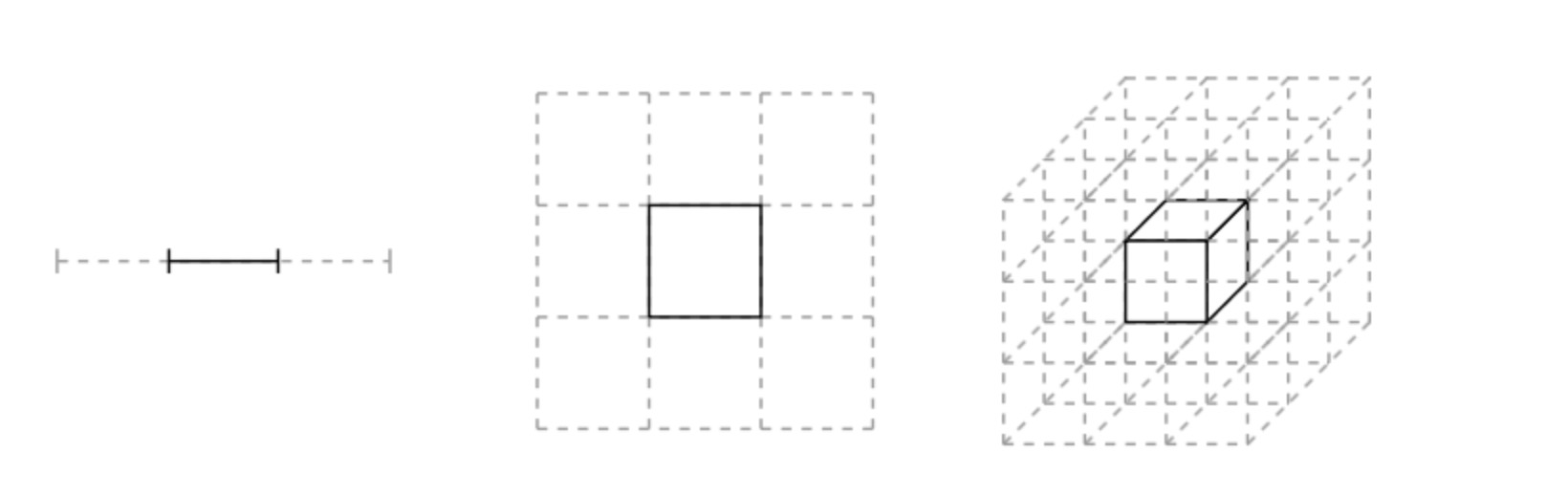where the centre-partitions combination to an integral goes from 1/3rd to 1/27th. Now suppose the mode of the distibution is contained in this partition: then its contribution to the integral is going down with dimensions. As the centre volume decreases, the outer volume increases, but this is in distribution tails, so we dont get much of a contribution from there either: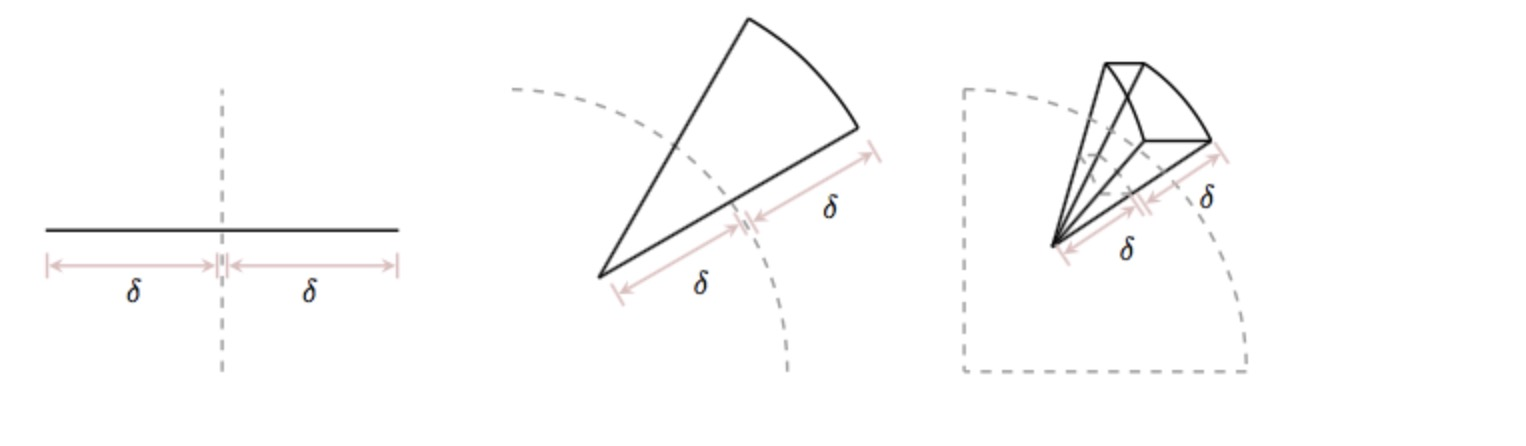It is the neighborhood between these extremes, called the typical set which our sampler must explore well. And to get a good rejection sampling majorizer for this becomes hard. Limitation of MH Although MH eventually converges to the true distributionP(x)$, we have no gaurantees as to when this will occur. • MH HAS A “burn-in” period: an initial number of samples are thrown away because they are not from the true distribution. • The “burn-in” period represents the un-converged part of the Markov Chain. • Knowing when to halt burin-in is an part. We will look at some techniques later in this lecture. Code of Metropolis Hasting Algorithm  def metropolis_hastings(p,q, qdraw, nsamp, xinit): samples=np.empty(nsamp) x_prev = xinit accepted=0 for i in range(nsamp): x_star = qdraw(x_prev) p_star = p(x_star) p_prev = p(x_prev) pdfratio = p_star/p_prev proposalratio = q(x_prev, x_star)/q(x_star, x_prev) if np.random.uniform() < min(1, pdfratio*proposalratio): samples[i] = x_star x_prev = x_star accepted +=1 else:#we always get a sample samples[i]= x_prev return samples, accepted # target function f = lambda x: 0.554*x*np.exp(-(x/1.9)**2) x = np.linspace(0,10,100) plt.plot(x, f(x), 'r') plt.grid('on') plt.title('The target function')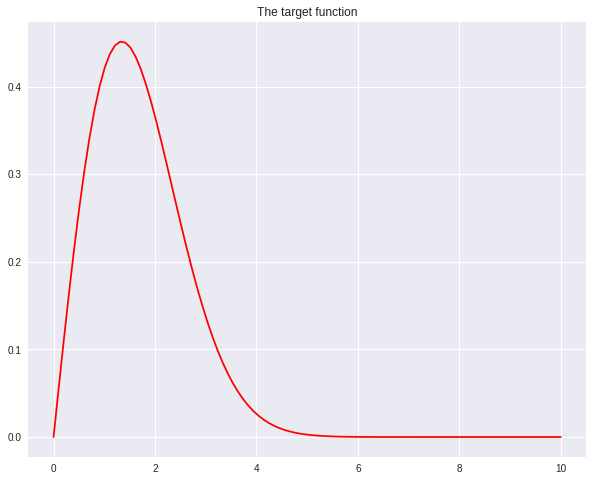from scipy.stats import gamma t=10.0 def gammapdf(x_new, x_old): return gamma.pdf(x_new, x_old*t, scale=1/t) def gammadraw(x_old): return gamma.rvs(x_old*t,scale=1/t) x_init = np.random.uniform() samps, acc = metropolis_hastings(f, gammapdf, gammadraw, 100000, x_init) # plot our sample histogram plt.hist(samps,bins=100, alpha=0.4, label=u'MCMC distribution', normed=True) somesamps=samps[0::20000] for i,s in enumerate(somesamps): xs=np.linspace(s-3, s+3, 100) plt.plot(xs, gamma.pdf(xs,s*t,scale=1/t),'k', lw=1) xx= np.linspace(0,10,100) plt.plot(xx, f(xx), 'r', label=u'True distribution') plt.legend() plt.xlim([0,10]) plt.show() print("starting point was ", x_init)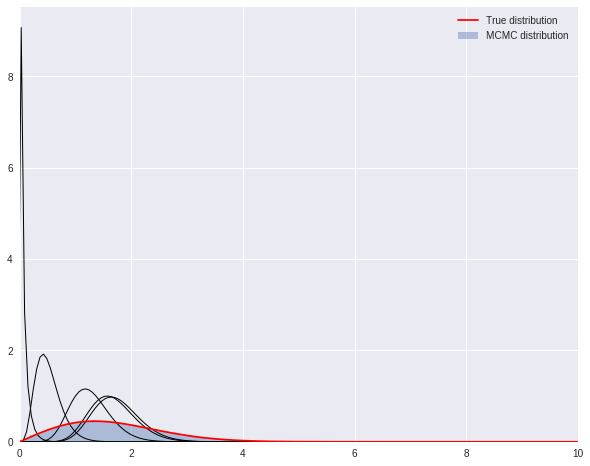### 2.4 Gibbs Sampling Gibbs sampling is an Markov Chain Monte Carlo algorithms that samples each random variables of a graphical, one at a tiem. It is a special case of the Metropolis-Hasting algorithm, which performs a biased random walk to explore distribution. It is assumed that$P(x)$is too complex while$P(x_{i}\vert x_{-i})$is tractable to work with. Algorithm 1. Let$x_{1},…,x_{n}$be the variables of the graphical model for which we are estimate the distrition. 2. Initialize starting values for$x_{1},…,x_{n}$. 3. At time step$t$: • Pick an arbitrary ordering of$x_{1},\cdots, x_{n}$(this can be arbitrary or random). • For each$x_{i}$in the order: Sample$x_{i}^{t}\sim P(x_{i}\vert x_{-i})$, where$x_{i}$is updated immediately by$x_{i}^{t}$(the new value will be used for the next sampling) 4. Repeat until convergence. Gibbs determined the energy states of gases at equilibrium by cycling through all the particles, drawing from each one of them conditionally given the enerygy levels of the others, taking the time average. How do we compute the conditional probability$P(x_{i}\vert x_{-i})$? (With Markov Blankets) $\begin{equation} P(x_{i}\vert x_{-i}) = P(x_{i}\vert MB(x_{i})) \end{equation}$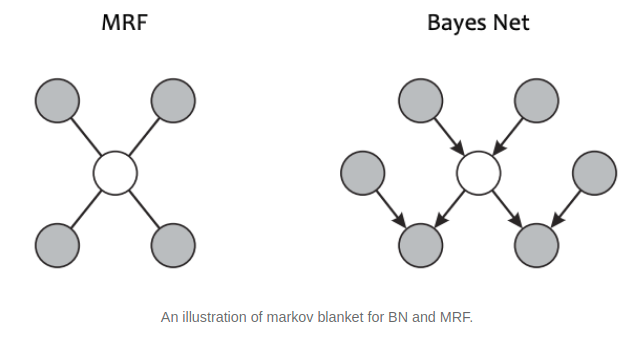For a Bayesian Network, the Markov Blanket of$x$is the set of parents, children and co-parents. For a Markov Random Field, the Markov Blanket of$x$is its immediate neighbors. A 2D Example The following figure illustrates Gibbs Sampling on two variables$(x_{1},x_{2})=x$.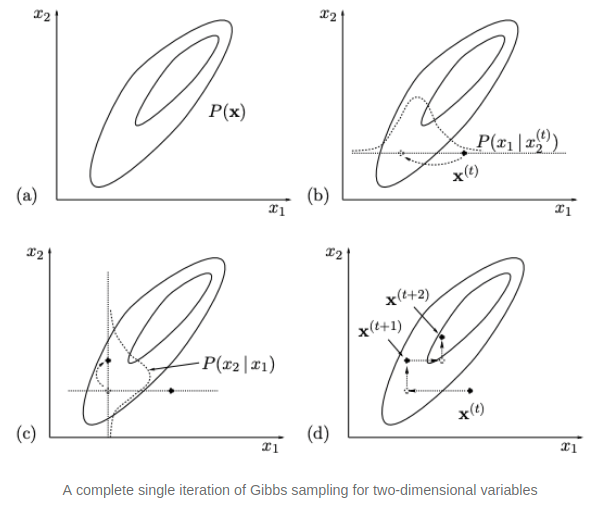On each iteration, we start from the current state$x^{(t)}$and$x_{1}$is sampled from conditional density$P(x_{1}\vert x_{2})$, with$x_{2}$fixed to$x_{2}^{(t)}$. Then$x_{2}$is sampled from conditional density$P(x_{2}\vert x_{1})$, with$x_{1}$fixed with$x_{1}^{(t+1)}$. This gives$x^{(t+1)}$and completes the iteration. ### 2.5 Gibbs and MH Algorithm Gibbs is the extension or a special case of MH Algorithm in high-dimensional space with accepted rate 1. The Gibbs Sampling proposal distribution is $\begin{equation} Q(x^{\prime}_{i}, x_{-i}\vert x_{i}, x_{-i}) = P(x^{\prime}_{i} \vert x_{-i}) \end{equation}$ Applying Metropolis-Hastings to this proposal, we find that samples are always accepted $\begin{equation} \begin{split} A(x_{i}^{\prime}, x_{-i}\vert x_{i}, x_{-i}) &=\min (1, \frac{P(x^{\prime}_{i}, x_{-i})Q(x_{i}, x_{-i}\vert x_{i}^{\prime}, x_{-i})}{P(x_{i}, x_{-i})Q(x_{i}^{\prime}, x_{-i}\vert x_{i}, x_{-i})}) \\ &= \min(1, \frac{p(x_{i}^{\prime})P(x_{i}\vert x_{-i})}{P(x_{i},x_{-i})P(x^{\prime}_{i}\vert x_{-i})}) \\ &=\min(1, \frac{P(x_{i}^{\prime}\vert x_{-i})P(x_{-i})P(x_{i}\vert x_{-i})}{P(x_{i}\vert x_{-i})P(x_{-i})P(x_{i}^{\prime}\vert x_{-i})}) \\ &=\min(1, 1) \\ &=1 \end{split} \end{equation}$ Code of Gibbs sampling $\begin{equation} f(x,y)=x^{2}\text{exp}(-xy^{2}-y^{2}-2*y-4*x) \end{equation}$ ## 3. Pratial Aspects of MCMC (1). How do we know if our proposal is any good? (Monitor the acceptance rate:) Choosing the proposal is a tradeoff. The ‘narrow’, low-variance proposals have high acceptance, but may take many iterations to explore$P(x)$fully because the proposed$x$are too close. The ‘wide’, high-variance proposals have the potential to explore much of$P(x)$, but many proposals are rejected which slows down the sampler. A good$Q(x^{\prime}\vert x)$proposes distant samples$x^{\prime}$with a sufficiently high acceptance rate.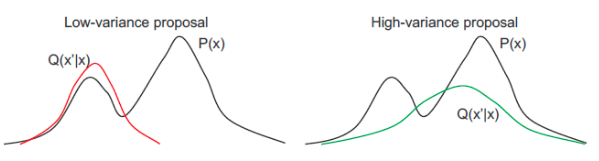Acceptance rate is the fraction of samples that MH accepts. A general guideline is proposals should have ~0.5 acceptance rate. If both$P(x)$and$Q(x^{\prime}\vert x)$are Gaussian, the optimal rate is$\sim 0.45$for$D=1$dimension and approaches$\sim 0.23$as$D$tends to infinity. (2). How do we know if our proposal is any good? – Autocorrelation function: MCMC chains always show autocorrelation (AC), because we are using the previous example to define the transition of the next example. (Note: AC means that adjacent samples in time are highly correlated.) We quantify AC with the autocorrelation fucntion of an r.v.x: $\begin{equation} R_{x})(k) = \frac{\sum_{t=1}^{n-k}(x_{t}-\bar{x})(x_{t+k}-\bar{x})}{\sum_{t=1}^{n-k}(x_{t}-\bar{x})^{2}} \end{equation}$ The first-order AC$R_{x}(1)$can be used to estimate the Sample size inflation Factor (SSIF): $\begin{equation} s_{x} = \frac{1+R_{x}}{1-R_{x}} \end{equation}$ If we took$n$samples with SSIF$s_{x}$, then the effective sample size is$\frac{n}{s_{x}}$. High autocorrelation leads to smaller effective sample size. We want proposals$Q(x^{\prime}\vert x)$with low correlation.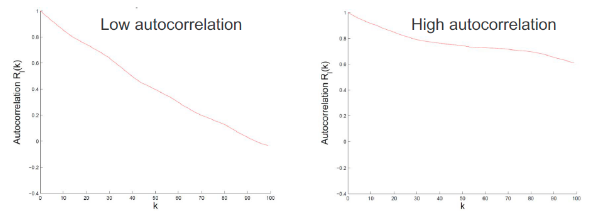(3). How do we know when to stop burn-in? – Plot the sample values vs time We can monitor convergence by plotting samples (of r.v.s) from multiple MH runs (chains). (Note: In practice, when people do MCMC, they usually start with multiple MCMC chains rather than one MCMC). If the chains are well-mixed (left), they are probably converged. If the chains are poorly-mixed (right), we should continue burn-in.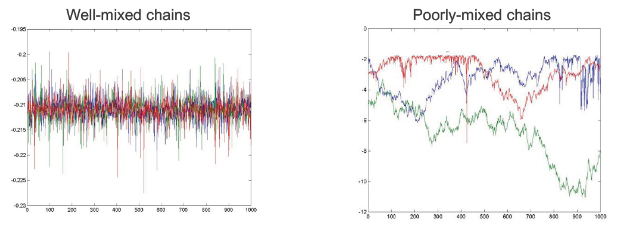(4). How do we know when to stop burn-in? – Plot the log-likelihood vs time Many graphical models are high-dimensional, so it is hard to visualize all r.v. chains at once. Instead, we can plot the complete log-likelihood vs. time. The complete log-likelihood is an r.v. that depends on all model r.v.s. Generall, the log-likelihood will climb, then eventually plateau.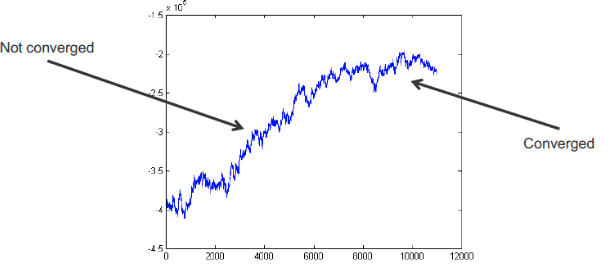## 4. Hamilton Monte Carlo One of the struggle people had in all vanilla MCMC methods is so called random walk behavior, which is caused by the proposed distribution. However, we want to propose prefered biased samples. How to impose the derivative (maybe likelihood function) into the proposal in a mathematically elegent fashion had became an important question. ### 4.1 Hamliton System Hamilton system is defined: $\begin{equation} H(p, x) = U(x) + K(p) \end{equation}$ where$x$is the position vector,$p$is the momentum vector.$U(x)$is the potential energy and$K(p)$stands for kinetic energy. One of key property of Hamilton system is $\begin{equation} \begin{split} \frac{\mathrm{d}x_{i}}{\mathrm{d}r} &= \frac{\partial H}{\partial p_{i}} \\ \frac{\mathrm{d}p_{i}}{\mathrm{d}t} &= -\frac{\partial H}{\partial x_{i}} \end{split} \end{equation}$ when we want to sample a target distribution, we can leverage on gradient methods by introducing more variables to an auxilliary distribution,$P_{H}(x, p)=\frac{e^{-E(x)-K(p)}}{Z_{h}}$. Thus, using Hamilton, we are able to define the change of state v.s. the gradient of a loss function over the change. (1). How to update: Euler’s Method There are multiple way to compute the$\delta$in the state as a function of teh gradient. The Euler’s Method directly estabilsh the change in$p$(momentum), and$q$(position) as a function of the loss.$$\begin{equation} \begin{split} p_{i}(t+\epsilon) &= p_{i}(t) + \epsilon\frac{\mathrm{d}p_{i}}{\mathrm{d}t}(t) = p_{i}(t) -\epsilon \frac{\partial U}{\parital q_{i}}(q(t)) q_{i}(t+\epsilon) &= q_{i}(t) +\epsilon \frac{\mathrm{d}q_{i}}{\mathrm{d}t}(t) = q_{i}(t) + \epsilon \frac{p_{i}(t)}{m_{i}} \end{split} \end{equation} (2). How to update: Leapfrog Method Leapfrog Method is prefered, because it alternates between the$p$and$q$to calculate the updates in a very controlled fashion. So behaviors like over shooting and under shooting can be avoided. $\begin{equation} \begin{split} p_{i}(t+\epsilon /2) &= p_{i}(t) -(\epsilon/2)\frac{\partial U}{\parital q_{i}}(q(t)) \\ q_{i}(t+\epsilon) &= q_{i}(t) +\epsilon \frac{p_{i}(t+\epsilon/2)}{m_{i}} \\ p_{i}(t+\epsilon) &= p_{i}(t+\epsilon/2) -(\epsilon/2)\frac{\partial U}{\partial q_{i}}(q(t+\epsilon)) \end{split} \end{equation}$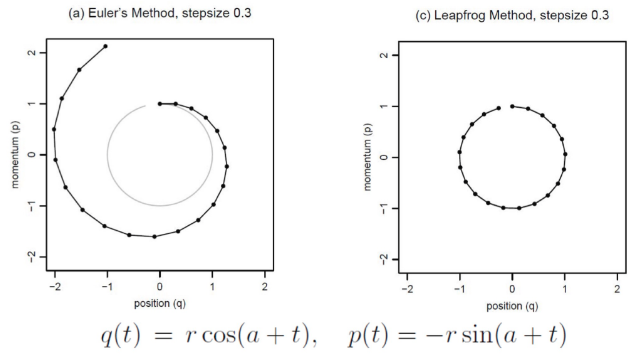### 4.2 MCMC from Hamiltonian Dynamics Let$q$be variable of interest,$p$is introduced as an auxiliary random variable in order to define the Hamiltonian. $\begin{equation} P(p,q) = \frac{1}{Z}\text{exp}(-U(q)/T)\text{exp}(-K(p)/TY) \end{equation}$ where$U(q)=-\log[\pi(q)L(q\vert D)]$and$K(p)=\sum_{i=1}^{d}\frac{p_{i}^{2}}{2m_{i}}$. Here it is a Bayesian setting where we have both the distribution of hidden states or the states of interest and also conditioned from priors.$U(q) = -\log [\pi (q) L(q\vert D)]$connects to the likelihhod, the gradient of which is not directly involved in the proposal of next qq . Then a accept/ reject critera is built based on the change of the Hamiltonian. ### 4.3 Langevin Dynamics Langevin Dynamics is special case of Hamiltonian. Instead of doing Leapfrog, Langevin does a more sophiscated update based on second-order updates of the sampling states. $\begin{equation} q^{\ast}_{i} =q_{i} -\frac{\epsilon^{2}}{2}\frac{\partial U}{\parital q_{i}}(q) + \epsilon p_{i} \end{equation}$$p_{i}^{\ast}=p_{i} - \frac{\epsilon}{2}\frac{\partial U}{\partial q_{i}}(q)-\frac{\epsilon}{2}\frac{\partial U}{\partial q_{i}}(q^{\ast})$.Even for a strange distribution with constrains on regions, this augmented optimization methods still deal with it. ## 5. Summary ### 5.1 Summary on MCMC • Markov Chain Monte Carlo methods use adaptive proposal$Q(x^{\prime}\vert x)$to sample from the true distribution$P(x)$. • Metropolis-Hasting allows you to specify any proposal$Q(x^{\prime}\vert x)$. Though choosing a good$Q(x^{\prime}\vert x)$carefully. • Gibbs sampling sets the proposal$Q(x^{\prime}\vert x)$to the conditional distribution$P(x^{\prime}\vert x)\$:
1. Acceptance rate is 1.
2. high acceptance rate entails slow exploration.
3. In fact, there are better MCCM algorithms for certain models.
• Knowing when to halt burn-in state.

### 5.2 Summary on HMC

• Using Hamiltonian, we are able to define the change of state v.s. the gradient of a loss function over the change.
• Hamiltonian Mento Carlo can improve acceptence rate and give better mixing by incorporating optimization based approaches to generate better proposals.
• Stochastic variants can be used to improve performance in large dataset scenarios.
• Hamiltonnian Mento Carlo may not be used for discrete variable